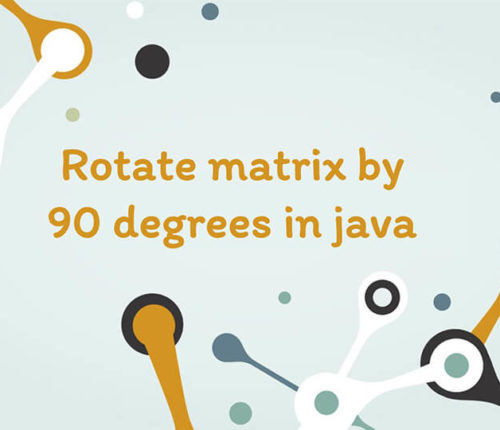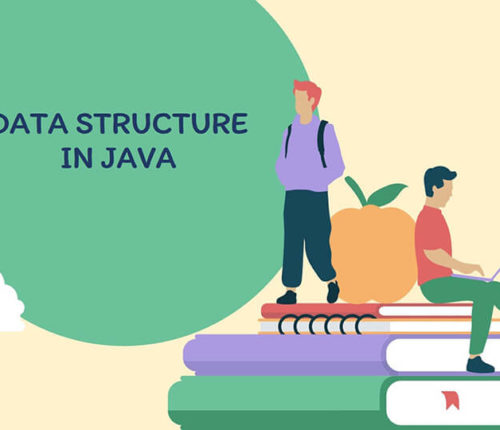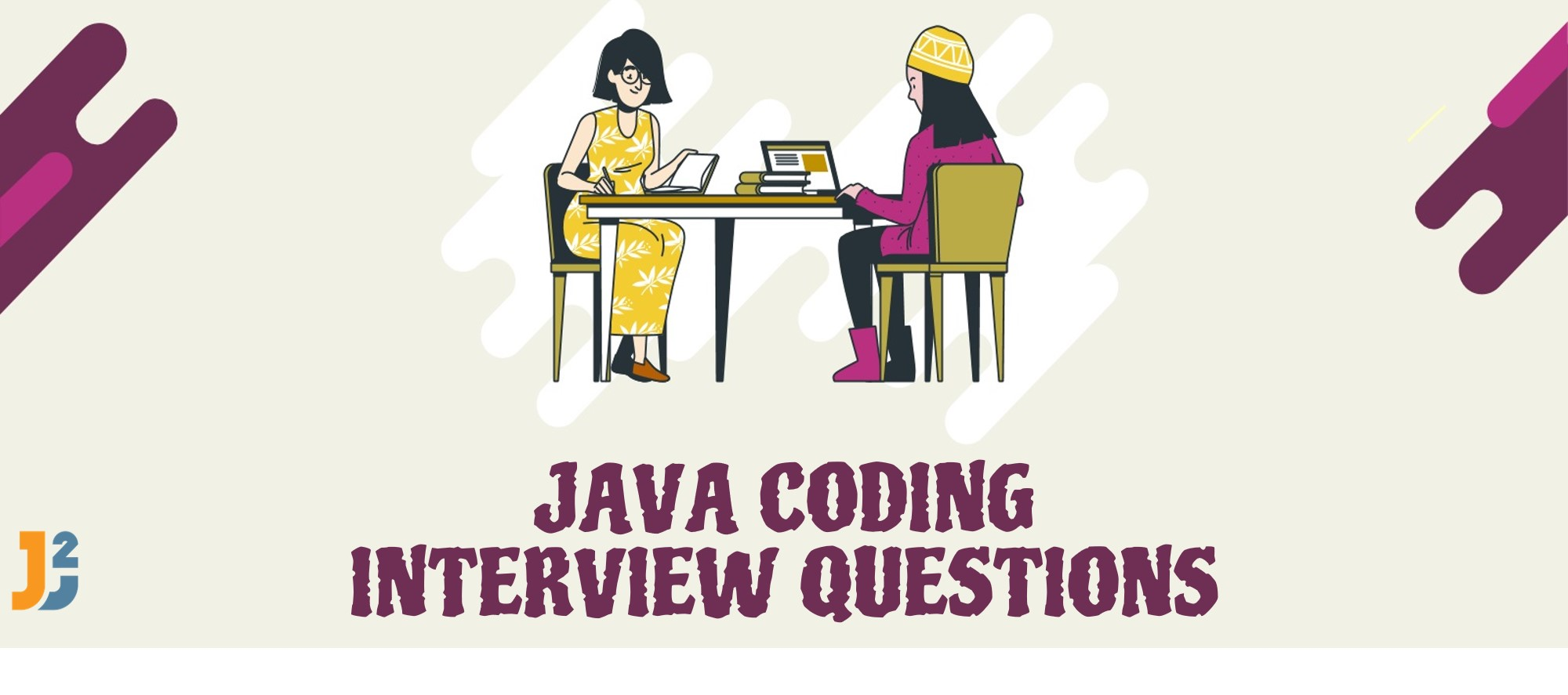# Find all Permutations of a String in java

In this post, we will see how to find all permutations of String in java.
We will use a very simple approach to do it.

Take out first character of String and insert into different places of permutations of remaining String recursively.

Lets say you have String as ABC.
So we take out A from ABC
First character =A and RemainingString = BC
As we are applying recursion here, we will find permutations of BC.
Take out B from BC.
First character= B and RemainingString = C
As we are applying recursion here, we will find permutations of C.
When we take out C, our String size becomes 0 and that is our base case here.
First character = C and RemainingString = “”
We insert C to differences indexes of Permutations  of RemainingString(“”), so we get permutation of C as C.
We insert B to different indexes of Permutations of remainingString(C), so we get BC and CB.
C: BC, CB
Now we insert A to different indexes in BC and CB.
BC : ABC , BAC , BCA
CB : ACB, CAB, CBA
so thats how we got all permutations of ABC.
It may look tricky but once you practice the solution, you will be able to understand it better.

### Java program to find all Permutations of String in java:

When you run above program, you will get following out:

## Related Posts

•20 May

### Rotate Matrix by 90 degrees in java

Table of ContentsClockwise or Right Rotate a MatrixAnti-Clockwise or Left Rotate a MatrixHow to Rotate Matrix K Times? In this article, we will look into another interesting problem related to 2D Arrays. Given a Matrix of N X N Dimension we have to Rotate matrix by 90 degrees. We will perform Rotation both Clockwise i.e. […]

•16 April

### Data Structures in java

•29 November

### Top 100+ Java coding interview questions

Table of ContentsStringQuestion 1 : How to reverse a String in java? Can you write a program without using any java inbuilt methods?Question 2 : Write a java program to check if two Strings are anagram in java?Question 3 : Write a program to check if String has all unique characters in java?Question 4 : […]

•18 April

### Minimum Number of Jumps to reach last Index

Table of ContentsProblemSolution If you want to practice data structure and algorithm programs, you can go through Java coding interview questions. In this post, we will see how to find Minimum Number of Jumps to reach last Index. Problem Given an array A of positive integers possibly zeroes, every index indicating the maximum length of a […]

•20 February

### Inorder Successor in a Binary Search Tree

Table of ContentsProblemSolutionAPPROACH – I :Approach – II:(i) If the target node has a right child :(ii) If the target node does not have a right child :Time Complexity Discussion If you want to practice data structure and algorithm programs, you can go through Java coding interview questions. In this post, we will see how to […]

•04 February

### LCA of a K-ary Tree in O(Sqrt(height))

Table of ContentsProblemSolution If you want to practice data structure and algorithm programs, you can go through Java coding interview questions. In this post, we will see about how to find Lowest Common Ancestor of a K-ary Tree in O(Sqrt(height)).We have already seen how to find LCA of n-ary tree in O(n) complexity. Problem Given a […]

## Subscribe to our newletter

Get quality tutorials to your inbox. Subscribe now.